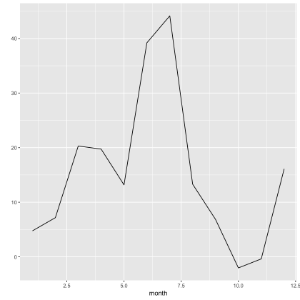# Using dbplot Package with tdplyr | Teradata Package for R - 16.20 - Using dbplot Package with tdplyr - Teradata R Package

## Teradata® R Package User Guide

Product
Release Number
16.20
Published
February 2020
Language
English (United States)
Last Update
2022-05-02
dita:mapPath
qbt1519078127352.ditamap
dita:ditavalPath
Generic_no_ie_no_tempfilter.ditaval

The dbplot package is a simple yet powerful open source package for visualizing computations in the database without bringing the data to the client. The APIs in the dbplot library can be implemented in R to add this feature to the tdplyr library.

The following examples use the "flights" dataset from the "nycflights13" package and the use cases from dbplot, to show how to use dbplot package with the tdplyr.

## Examples Prerequisite

You must have the "ggplot2" and "dbplot" packages, and the "nycflights13" data package on your R client first. And prepare the data for plotting.

`library(ggplot2)`
`install.packages("dbplot", quiet = TRUE)`
`library(dbplot)`
The db_compute_boxplot and dbplot_boxplot functions from the dbplot package are not supported as their internal SQL translations are incompatible with Teradata.
Install the "nycflights13" package on your R client, if it is not already installed. And load the library.
`install.packages('nycflights13', quiet = TRUE)`
`library(nycflights13)`
Filter a subset of the data.
`flights_tibble <- as.data.frame(nycflights13::flights)%>% filter(dep_time < 600)`
Make sure you do not already have a table named 'flights' in the default database. Create the table and check its content.
`copy_to(con, flights_tibble, "flights")`
`flights <- tbl(con, "flights")`
`flights`
```        year month   day dep_time sched_dep_time dep_delay arr_time sched_arr_time
<int> <int> <int>    <int>          <int>     <dbl>    <int>          <int>
1  2013     1     1      542            540         2      923            850
2  2013     1     1      554            600        -6      812            837
3  2013     1     1      554            558        -4      740            728
4  2013     1     1      555            600        -5      913            854
5  2013     1     1      557            600        -3      838            846
6  2013     1     1      558            600        -2      753            745
7  2013     1     1      557            600        -3      709            723
8  2013     1     1      544            545        -1     1004           1022
9  2013     1     1      533            529         4      850            830
10  2013     1     1      517            515         2      830            819
# ... with more rows, and 11 more variables: arr_delay <dbl>, carrier <chr>,
#   flight <int>, tailnum <chr>, origin <chr>, dest <chr>, air_time <dbl>,
#   distance <dbl>, hour <dbl>, minute <dbl>, time_hour <dttm>```

## Use dbplot_histogram to plot Histogram

Remove NAs to avoid warning messages and plot the histogram of the flights data.
`flights %>% filter(!is.na(arr_time)) %>% dbplot_histogram(arr_time)`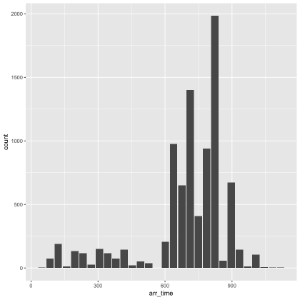Plot the histogram of the flights data with a custom bin width.
`flights %>% filter(!is.na(arr_time)) %>% dbplot_histogram(arr_time, binwidth = 200)`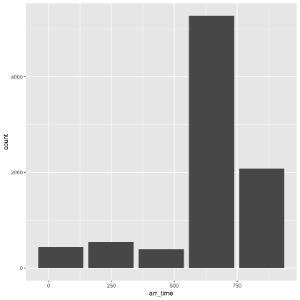Plot the histogram of the flights data with custom labels on the plot.
```flights %>% filter(!is.na(arr_time)) %>%
dbplot_histogram(arr_time) +
labs(title = "Flights - Arrival Time") +
theme_light()#also changing the theme```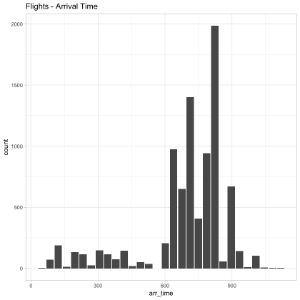## Use dbplot_raster to plot Raster Graphics

To visualize two continuous variables with millions or billions of dots representing the intersections of the two variables, you can use Raster plot. It concentrates the intersections into squares that are easy to parse visually.

A Raster plot basically does the same as a Histogram. It takes two continuous variables and creates discrete two-dimensional bins represented as squares in the plot. It then determines either the number of rows inside each square or processes some aggregation, like an average.
The default fill for a pixel is count(), it is the number of entries in each intersection square.
Remove NAs to avoid warning messages and plot the raster of the flights data.
```flights %>%
filter(!is.na(arr_delay)) %>%
dbplot_raster(arr_delay, dep_delay)```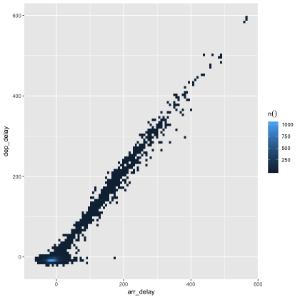Pass an aggregation formula that can run inside the database and plot the raster of the flights data.
```flights %>%
filter(!is.na(arr_delay)) %>%
dbplot_raster(arr_delay, dep_delay, fill = mean(distance, na.rm = TRUE))```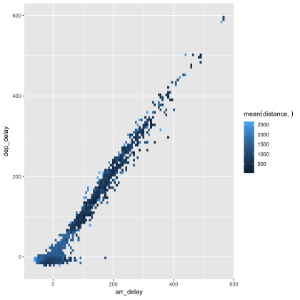Use the resolution argument to increase or decrease for more or less, and plot the raster. The default for resolution is 100.
```flights %>%
filter(!is.na(arr_delay)) %>%
dbplot_raster(arr_delay, dep_delay, mean(distance, na.rm = TRUE), resolution = 500)```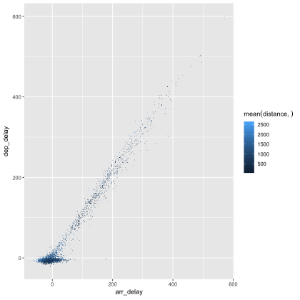## Use dbplot_bar to plot Bar graph

Default to a tally() of each value in a discrete variable to plot the bar graph.
```flights %>%
dbplot_bar(origin)```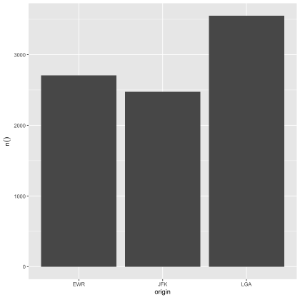Pass a formula that will be operated for each value in the discrete variable and plot the bar graph.
```flights %>%
dbplot_bar(origin, mean(dep_delay, na.rm = TRUE))```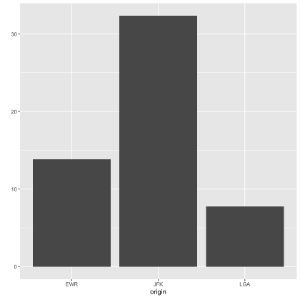## Use dbplot_line to plot Line graph

Default to a tally() of each value in a discrete variable to plot the line graph.
```flights %>%
dbplot_line(month)```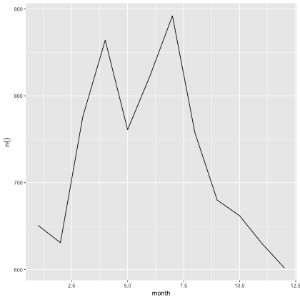Pass a formula that will be operated for each value in the discrete variable and plot the line graph.
```flights %>%
dbplot_line(month, mean(dep_delay, na.rm = TRUE))```